# Matlab群体智能优化算法之巨型睡莲优化算法(VAO)

Matlab群体智能优化算法之巨型睡莲优化算法(VAO)

• 仅记录学习过程，如有侵权，联系删除
• 本文所用墨滴模板为slandarer创作

• 灵感来源
• VAO算法
• 伪代码
• 评价和结果
• 写在最后

## 灵感来源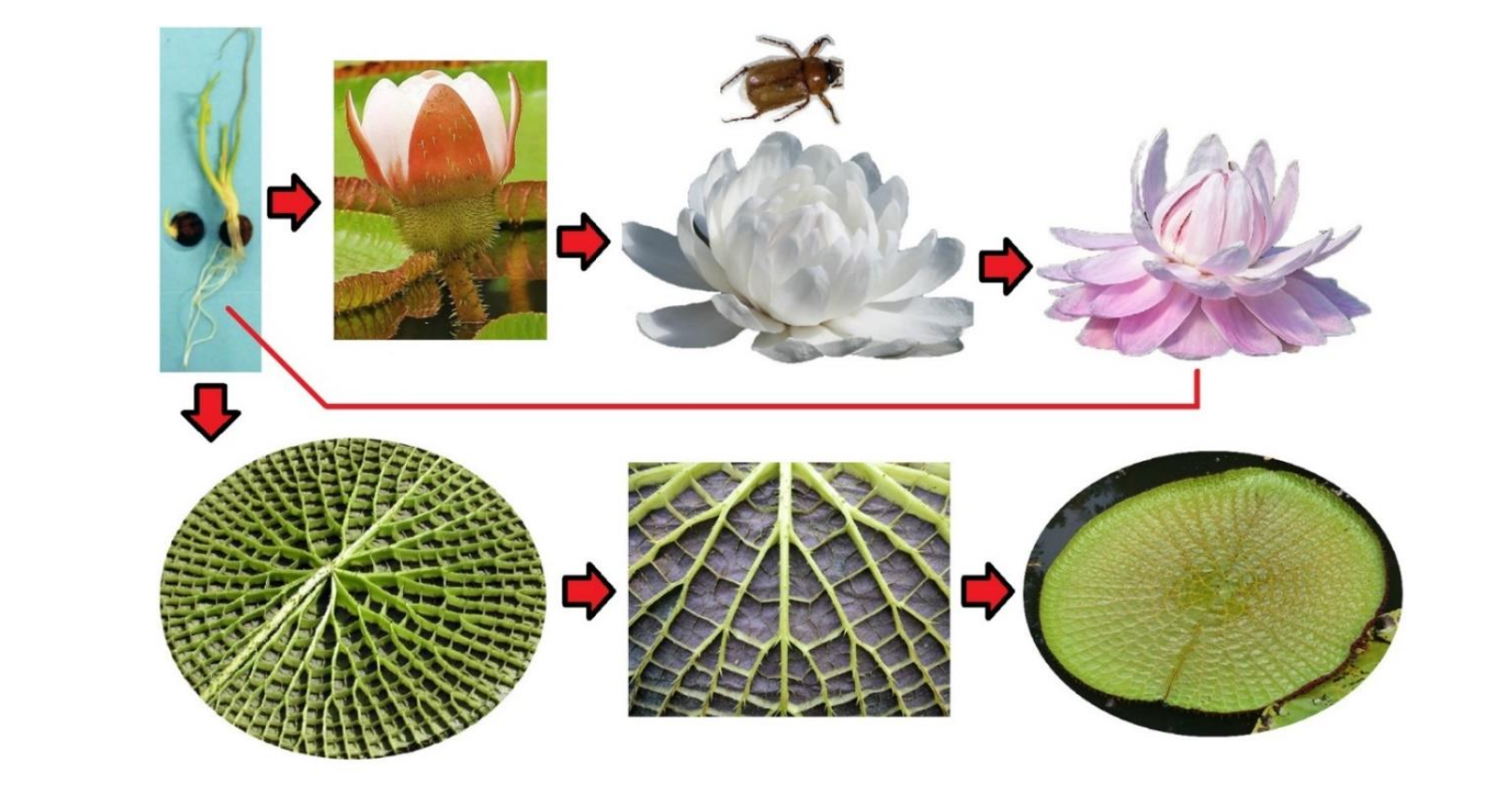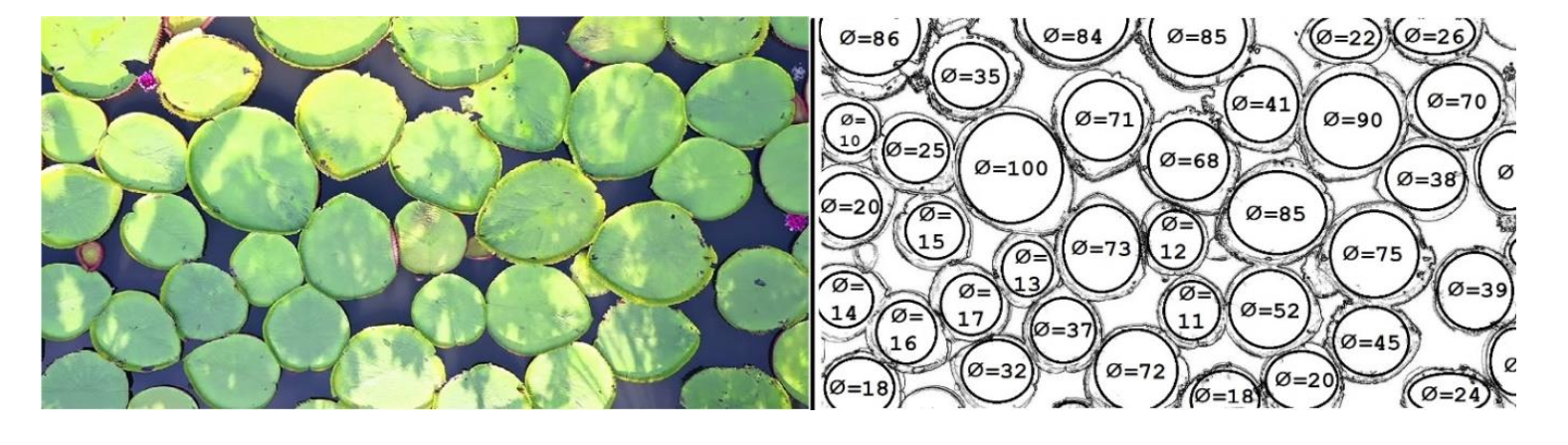## VAO算法

VOA算法关注的是初始种群(叶子和花)的分布以及它们在表面上响应的传播力。该算法主要是一种基于蜂群局部搜索的元启发式算法，因此唯一的缺点是有可能陷入局部最优。

• $\phi$ ：直径
• λ \lambda ：优化任务，本质上就是让直径越来越大。睡莲通过用上升的力量和脊柱强行置换彼此所能获得的空间大小，从而增大其直径。这种竞争被成为内部竞争，用 λ \lambda 表示。
• $\omega ：睡莲生长存在三个常见的困难。花内甲虫的死亡，甲虫不受粉或者授粉不当，以及温度降低。所有这些因素都有可能对这个过程不利，我们在这里把它们都成为 ：睡莲生长存在三个常见的困难。花内甲虫的死亡，甲虫不受粉或者授粉不当，以及温度降低。所有这些因素都有可能对这个过程不利，我们在这里把它们都成为 \omega 。显然， 。显然， \omega$的值越大，表明植物越弱。
• ψ \psi ：害虫，如睡莲蚜虫，也可以通过吃植物的叶子和在叶子上制造洞来伤害植物。本文用 ψ \psi 来代表这种威胁值。 ψ \psi 的值越低，对植物生长和扩张越有利。
• μ \mu ：最后，当池塘里的甲虫用另一种睡莲给花授粉时，就会发生突变。这种现象被成为“杂交突变”，用 μ \mu 表示。这种突变可以在正反两个方向上发生，每一代的比率为0.2%。
• α \alpha ：最大和最强的叶子时最好的，用 α \alpha 表示。

## 伪代码

V A O = ∑ i = 1 n ∑ j = 1 n ( x i j [ ∅ i j , λ i j ] + ψ + ω ) ∗ ( μ ) V A O=\sum_{i=1}^n \sum_{j=1}^n(x i j[\varnothing i j, \lambda \mathrm{ij}]+\psi+\omega) *(\mu)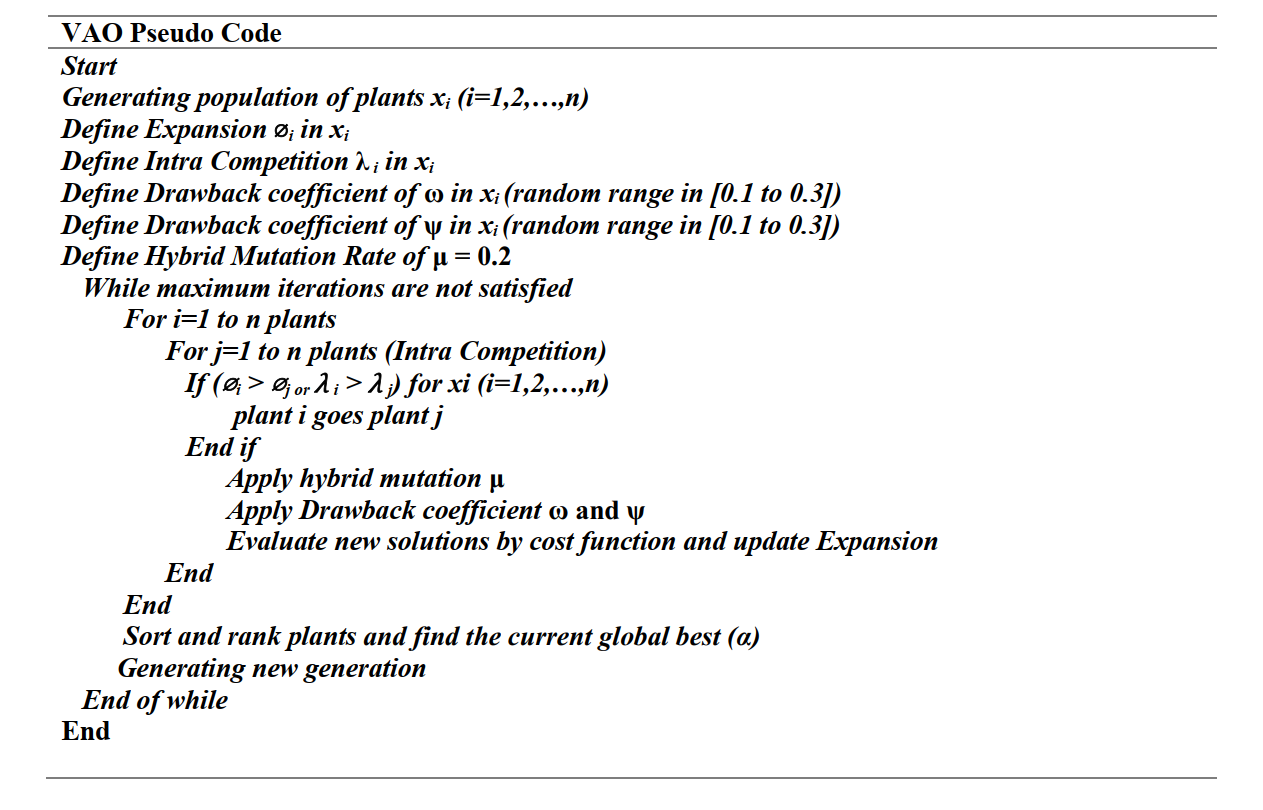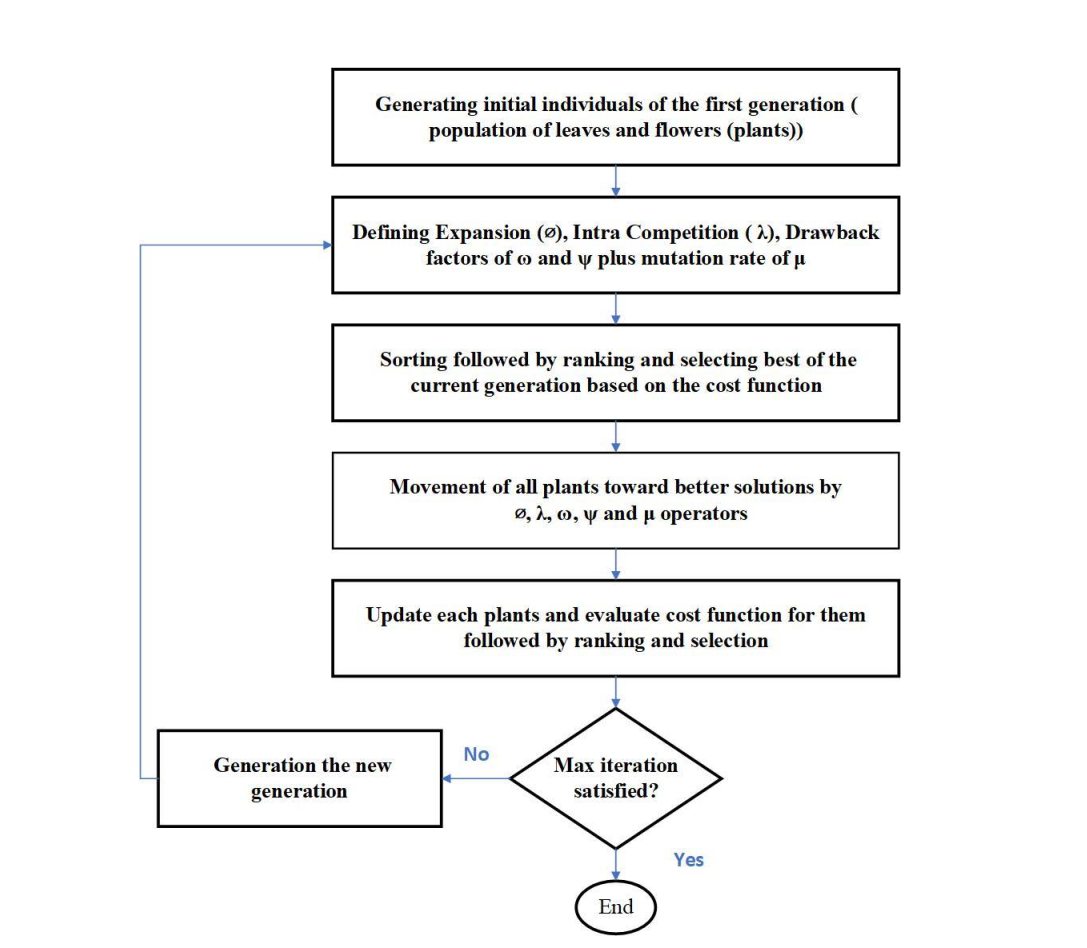• 加粗字体：MATLAB
• 斜体：MATLAB

## 评价和结果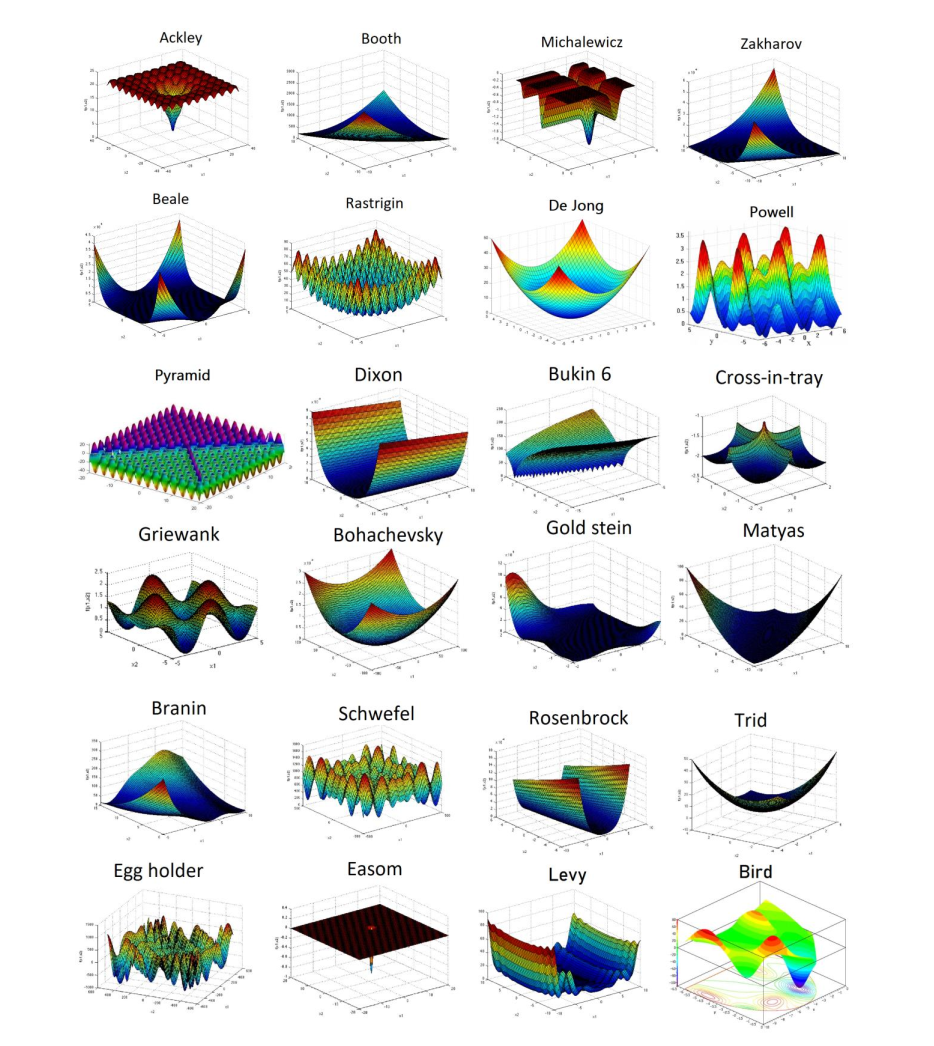http://www.sfu.ca/~ssurjano/index.html

KaTeX parse error: Expected 'EOF', got '_' at position 11: \text{new_̲x}=x_i+\psi * e…

μ = μ ∗ μ d a m p \mu = \mu*\mu_{damp} ;
μ d a m p = 0.98 \mu_{damp} = 0.98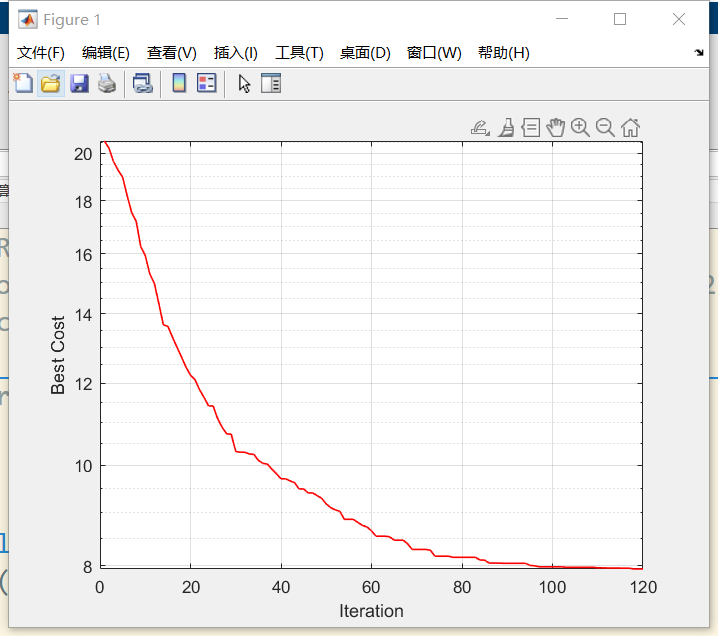Ackley函数，给了一个睡莲的分布图，和原文中的图9应该是对应的。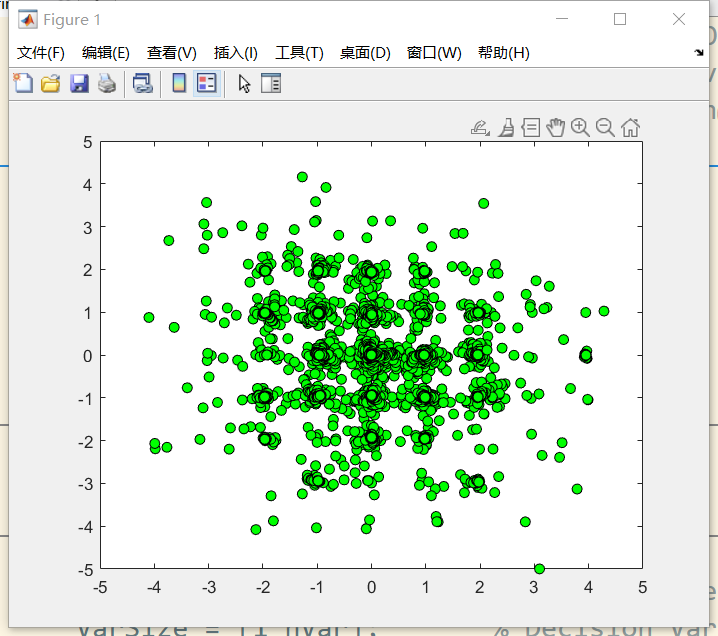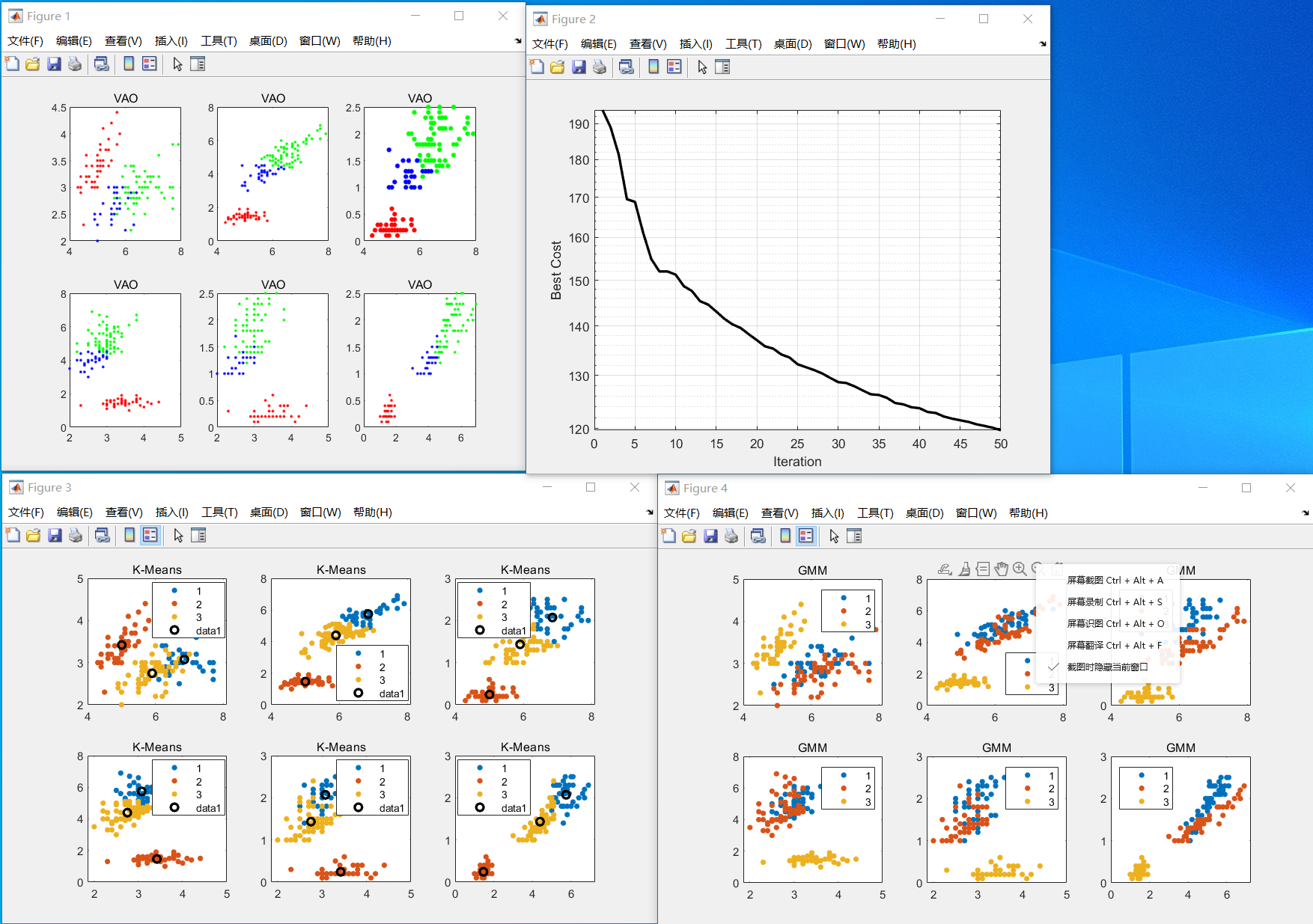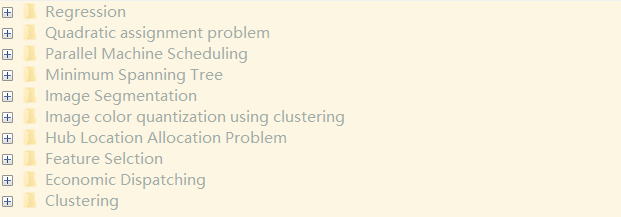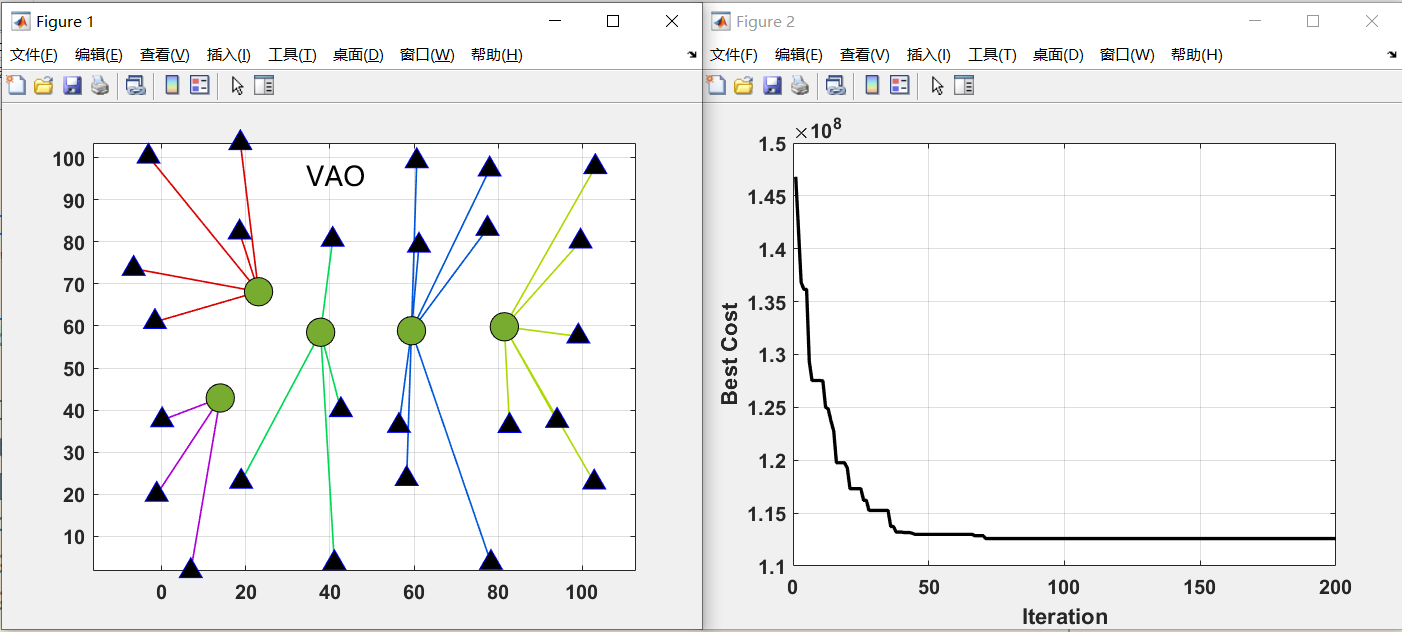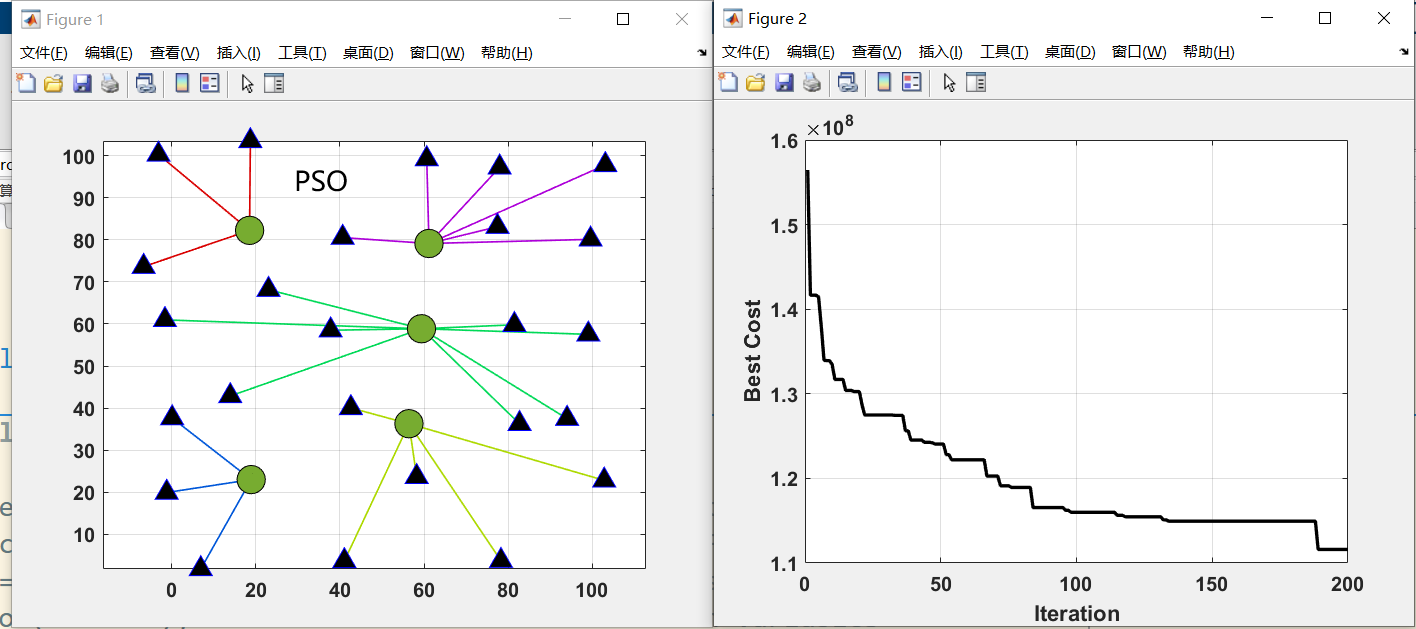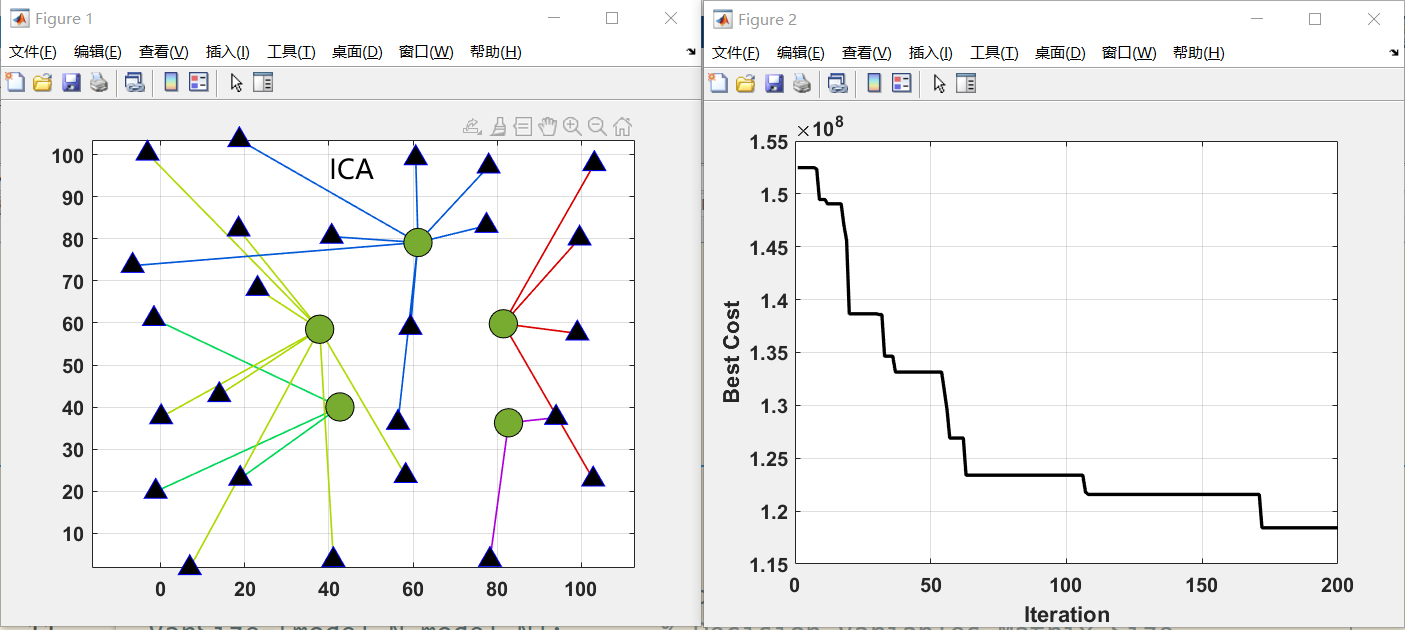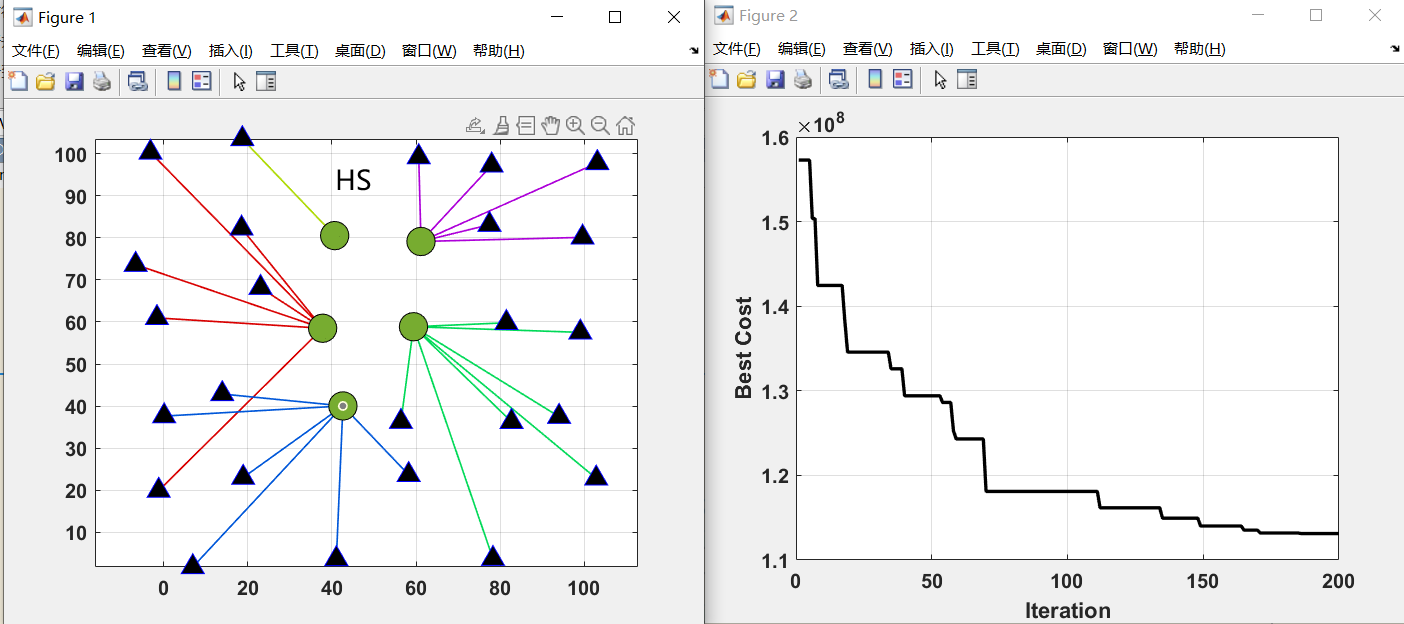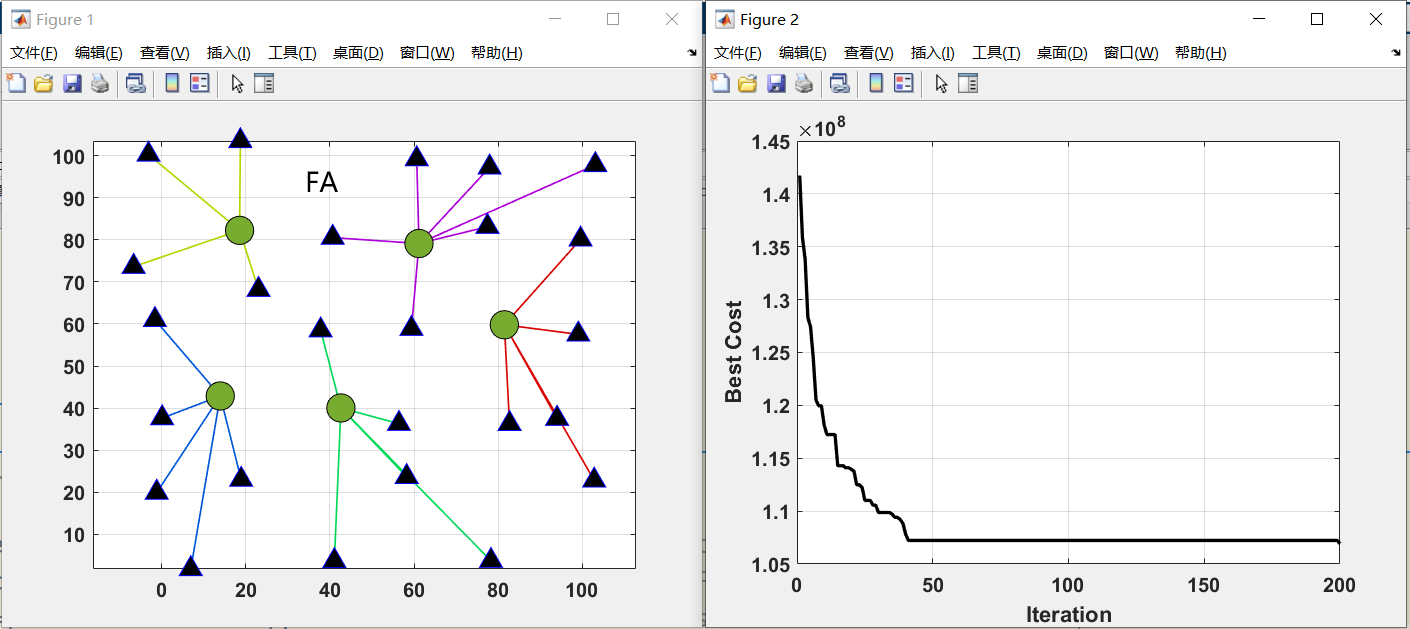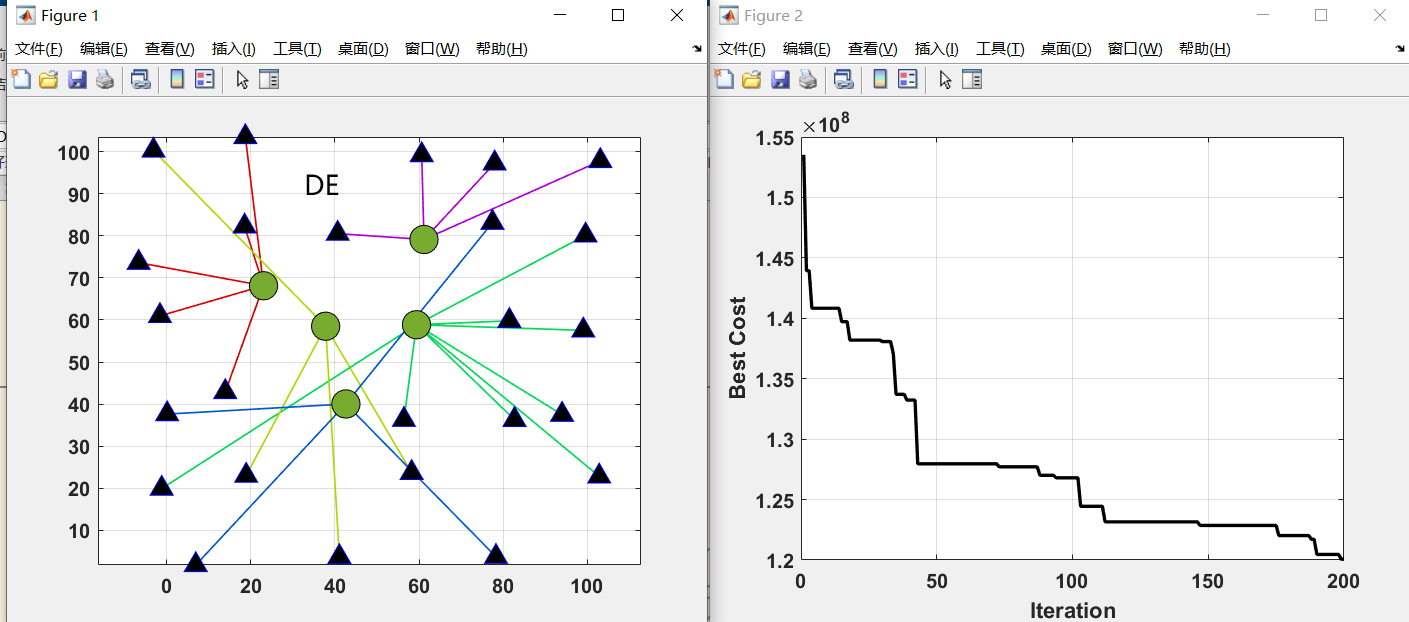## 写在最后

mathworks用QQ邮箱可以免费注册，无需科学上网。

Seyed Muhammad Hossein Mousavi (2023). Victoria Amazonica Optimization VAO Algorithm (https://github.com/SeyedMuhammadHosseinMousavi/Victoria-Amazonica-Optimization-VAO-Algorithm), GitHub. 检索来源 2023/3/29.